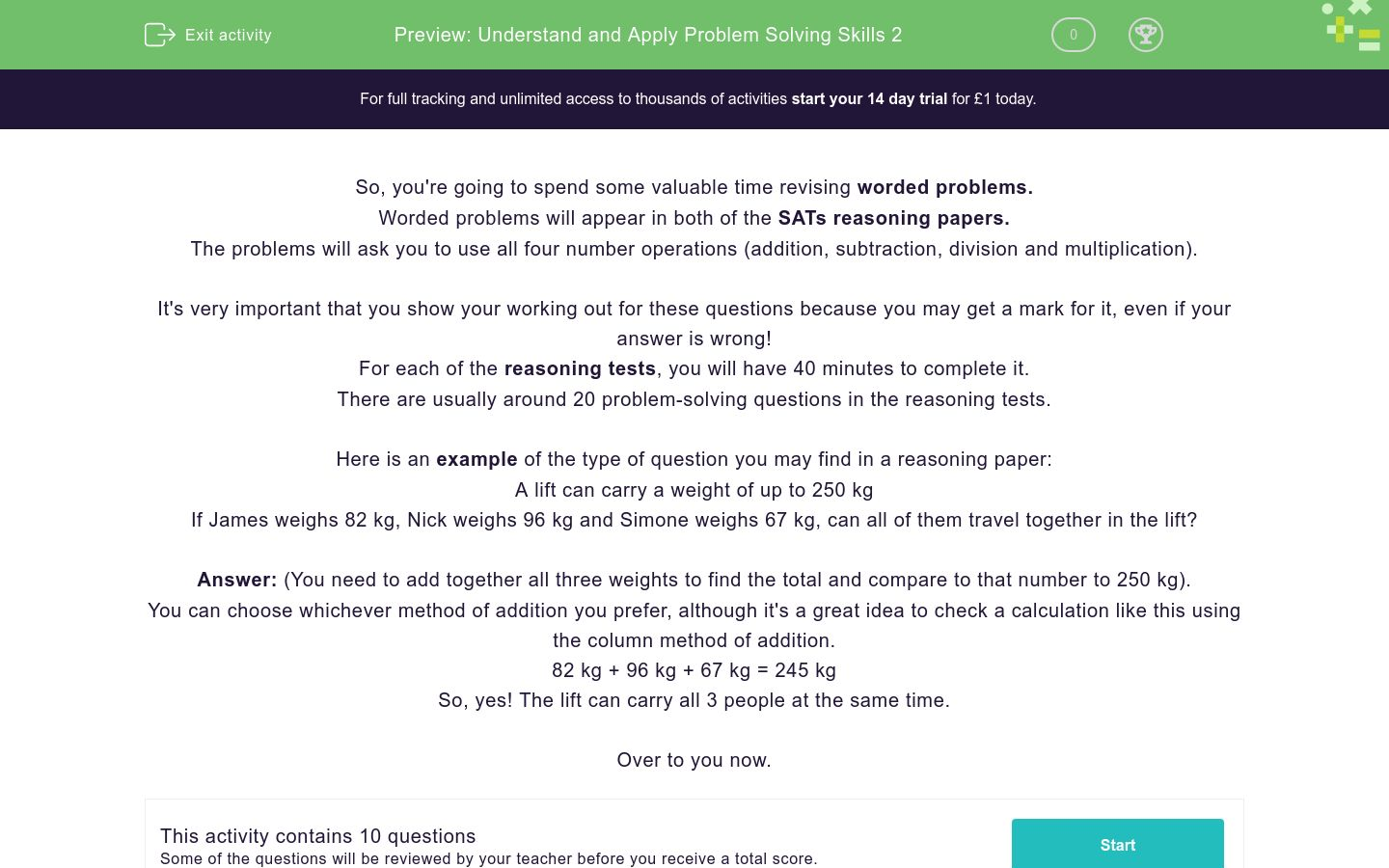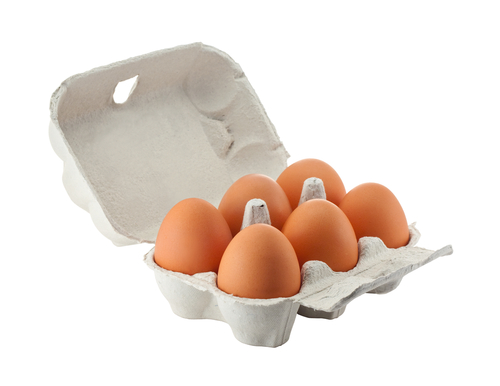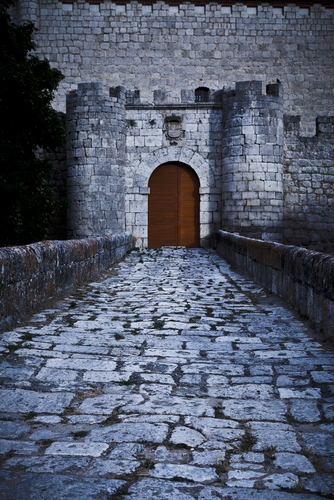# Understand and Apply Problem Solving Skills 2

In this worksheet, students will be asked to solve a range of worded problems, using all four operations. These types of problems appear in the two reasoning papers and the pupil is expected to have sufficient written proof to support each method required.Key stage:  KS 2

Curriculum topic:   Exam-style Questions: SATs Maths

Curriculum subtopic:   Exam-Style Questions: Worded Problems

Difficulty level:### QUESTION 1 of 10

So, you're going to spend some valuable time revising worded problems.

Worded problems will appear in both of the SATs reasoning papers.

The problems will ask you to use all four number operations (addition, subtraction, division and multiplication).

It's very important that you show your working out for these questions because you may get a mark for it, even if your answer is wrong!

For each of the reasoning tests, you will have 40 minutes to complete it.

There are usually around 20 problem-solving questions in the reasoning tests.

Here is an example of the type of question you may find in a reasoning paper:

A lift can carry a weight of up to 250 kg

If James weighs 82 kg, Nick weighs 96 kg and Simone weighs 67 kg, can all of them travel together in the lift?

Answer: (You need to add together all three weights to find the total and compare to that number to 250 kg).

You can choose whichever method of addition you prefer, although it's a great idea to check a calculation like this using the column method of addition.

82 kg + 96 kg + 67 kg = 245 kg

So, yes! The lift can carry all 3 people at the same time.

Over to you now.

There are 8 pencils in a box.

How many pencils does the school buy?Priya is saving her pocket money.

She saves £2.50 for a week.

How much has she saved after 12 weeks?

£20

£32

£30

£40

A spoonful of medicine measures at 5 ml.

How many spoonfuls can you get from a 400 ml bottle?A box can hold 6 eggs.

How many boxes are needed to hold 63 eggs?There are 68 children at a tennis club.

36 are boys.

How many are girls? (2 marks)

Explain how you found the answer.A can of coke costs 75 p.

How much does it cost to buy one for yourself and 5 friends?If a can of coca-cola is 79p a can and pizza £1.20 a slice, how much is it for 3 cokes and 3 slices of pizza?Adults £8.50 Children £5.25 OAPs £4.75

Above is the price list for entry to Warwick Castle.

How much will it cost for 4 adults to enter the castle?From the previous question, we know it costs £34 for 4 adults to enter the castle.

How much change will they get from a £50 note?Mrs Taylor's class have 240 exercise books at the start of the year.

12 children use 4 books each.

11 children use 5 books each.

How many books are left at the end of the year?• Question 1

There are 8 pencils in a box.

How many pencils does the school buy?EDDIE SAYS
Positive start if you calculated 176! This question required the multiplication 22 x 8 = 176. In the test, remember to show either column multiplication, partitioning or grid method for this type of multiplication calculation. Remember you may still get a mark for the working out, even if the answer is wrong.
• Question 2

Priya is saving her pocket money.

She saves £2.50 for a week.

How much has she saved after 12 weeks?

£30
EDDIE SAYS
How did you find this question? 12 x £2.50 = £30 There are different ways to solve this. A straightforward method is by using partitioning: 12 x £2 = £24 12 x £0.50 = £6 £24 + £6 = £30
• Question 3

A spoonful of medicine measures at 5 ml.

How many spoonfuls can you get from a 400 ml bottle?80
EDDIE SAYS
80 spoons can be taken from a 400 ml bottle. To solve this one, you had to carry out the number sentence: 400 ÷ 5 = 80. Using known times table facts can help solve this too: 40 ÷ 5 = 8 400 ÷ 5 = 80.
• Question 4

A box can hold 6 eggs.

How many boxes are needed to hold 63 eggs?EDDIE SAYS
Eggscellent work if you wrote 11! To 'crack' this one (see what I did there?!) you had to complete: 63 ÷ 6 = 10 remainder 3. We can't use 10, remainder 3 boxes so we have to round up and use 11 boxes.
• Question 5

There are 68 children at a tennis club.

36 are boys.

How many are girls? (2 marks)

Explain how you found the answer.• Question 6

A can of coke costs 75 p.

How much does it cost to buy one for yourself and 5 friends?EDDIE SAYS
Did you work out that 75p x 6 = 450p? Then, we have to convert this to pounds by dividing by 100 as there are 100p in £1. 450p ÷ 100 = £4.50 You are over halfway through this activity- keep going.
• Question 7

If a can of coca-cola is 79p a can and pizza £1.20 a slice, how much is it for 3 cokes and 3 slices of pizza?EDDIE SAYS
Don't worry if you found this tricky, it is definitely a challenge! Step 1: Cost of cola = 3 x 79p = £2.37 Step 2: Cost of pizza = 3 x £1.20 = £3.60 Step 3: Add both amounts together = £2.37 + £3.60 = £5.97.
• Question 8
 Adults £8.50 Children £5.25 OAPs £4.75

Above is the price list for entry to Warwick Castle.

How much will it cost for 4 adults to enter the castle?EDDIE SAYS
This is a tough one! £8.50 x 4 = £34 Partitioning is a great way to solve a question like this: £8.00 x 4 = £32 £0.50 x 4 = £2.00 Add together £32 + £2 = £34
• Question 9

From the previous question, we know it costs £34 for 4 adults to enter the castle.

How much change will they get from a £50 note?EDDIE SAYS
This is a nice, straightforward question. £50 - £34 = £16.
• Question 10

Mrs Taylor's class have 240 exercise books at the start of the year.

12 children use 4 books each.

11 children use 5 books each.

How many books are left at the end of the year?137
EDDIE SAYS
Did you read this question carefully? There were a few steps needed to find the answer. Step 1 12 x 4 = 48 books used Step 2 11 x 5 = 55 books used Step 3 Add total used together = 48 + 55 = 103 Step 4 Subtract amount used from original amount = 240 - 103 = 137 There are 137 books left. Great perseverance maths-whiz, you're now more prepared for the SATs reasoning papers.